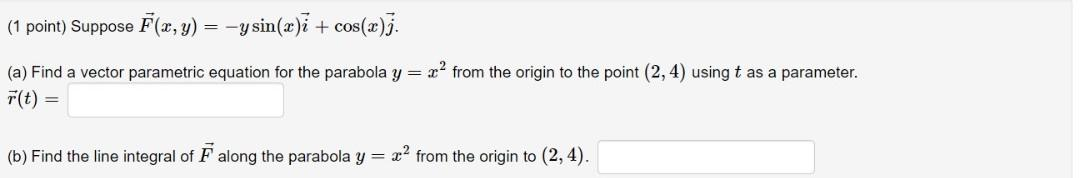### Create an Account

Home / Questions / (1 point) Suppose F(x, y) = -ysin(x)i + cos(x)]. (a) Find a vector parametric equation for...

# (1 point) Suppose F(x, y) = -ysin(x)i + cos(x)]. (a) Find a vector parametric equation for the parabola y = r2 from the origin to the point (2, 4) using t as a parameter. F(t) = (b) Find the line inte

(1 point) Suppose F(x, y) = -ysin(x)i + cos(x)]. (a) Find a vector parametric equation for the parabola y = r2 from the origin to the point (2, 4) using t as a parameter. F(t) = (b) Find the line integral of F along the parabola y = x2 from the origin to (2, 4).Apr 14 2021 View more View LessSubscribe To Get Solution